### Nonparametric Regression

Regression models that suppose a parametric form express the mean of an observation as a function of regressor variables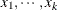and parameters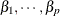: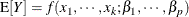Nonparametric regression techniques not only relax the assumption of linearity in the regression parameters, but they also do not require that you specify a precise functional form for the relationship between response and regressor variables. Consider a regression problem where the relationship between response Y and regressor X is to be modeled. It is assumed that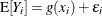, where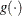is an unspecified regression function. Two primary approaches in nonparametric regression modeling are as follows:

• approximate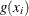locally by a parametric function constructed from information in a local neighborhood of• approximate the unknown functionby a smooth, flexible function and determine the necessary smoothness and continuity properties from the data

The SAS/STAT procedures LOESS, GAM, and TPSPLINE fit nonparametric regression models by one of these methods.